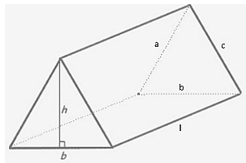# Volume of a Triangular Prism Formula

## Volume of a Triangular Prism Formulas - Definition & Examples

Calculating the Volume of a Triangular Prism
A prism is a solid object that has two congruent faces on either end joined by parallelogram faces laterally. A right prism has rectangular faces instead of parallelogram ones. Both these types of prisms have the same formula for volume. The following diagram shows a right triangular prism and its dimensions.The volume of any prism is equal to the product of its cross section (base) area and its height (length).
$V = BA \times l$
In the case of a triangular prism, the base area is the area of the triangular base, which can be calculated using Heron’s formula (if the lengths of the sides of the triangle are known) or by using the standard area of a triangle formula (if the lengths of a side of the triangle and its corresponding altitude are known).
$\begin{gathered} BA = \sqrt {s\left( {s - a} \right)\left( {s - b} \right)\left( {s - c} \right)} \hfill \\ s = \frac{{\left( {a + b + c} \right)}}{2} \hfill \\ \end{gathered}$
We must always take care of the units of measurement in mathematics. For example, if we want the volume in m3, then we need to calculate the base area in ${m^2}$ and the length in $m$.
Let’s look at an example to see how to use the formula!

Question: A prism has triangular ends whose sides are 3 cm , 4 cm and 5 cm .If its volume is 84 cm3 then find its length.
Solution:
$\begin{gathered} a = 3\,cm,\,\,b = 4\,cm,\,\,c = 5\,cm \hfill \\ s = \frac{{\left( {a + b + c} \right)}}{2} = \frac{{\left( {3 + 4 + 5} \right)}}{2} = \frac{{12}}{2} = 6\,cm \hfill \\ \end{gathered}$
$\begin{gathered} BA = \sqrt {s\left( {s - a} \right)\left( {s - b} \right)\left( {s - c} \right)} \hfill \\ \quad \,\, = \sqrt {6\left( {6 - 3} \right)\left( {6 - 4} \right)\left( {6 - 5} \right)} \hfill \\ \quad \,\, = \sqrt {6 \times 3 \times 2 \times 1} = \sqrt {36} = 6\,c{m^2} \hfill \\ \end{gathered}$
$\begin{gathered} V = BA \times l \hfill \\ l = \frac{V}{{BA}} = \frac{{84\,c{m^3}}}{{6\,c{m^2}}} = 14\,cm \hfill \\ \end{gathered}$
Why don’t you try to solving a problem yourself to see if you have mastered the formula!

Question: A triangular prism is such that one of the sides of its triangular faces and its corresponding height are equal in length (say x cm). The length and the volume of the prism are respectively equal to 10 cm and 80 cm3. Find the value of x.
Options:
(a) 6 cm
(b) 4 cm
(c) 8 cm
(d) none of these
$\begin{gathered} BA = \left( {\frac{1}{2}} \right) \times x \times x = \frac{{{x^2}}}{2} \hfill \\ V = BA \times l \hfill \\ BA = \frac{V}{l} \hfill \\ \frac{{{x^2}}}{2} = \frac{{80}}{{10}} = 8 \hfill \\ {x^2} = 16 \hfill \\ x = 4\,cm \hfill \\ \end{gathered}$